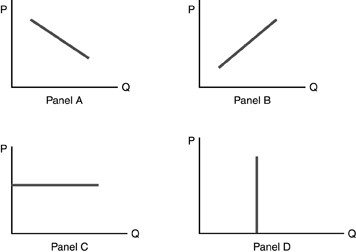• FAQ
• Contact/ Homework Answers / Economics / 91) A market demand schedule for a product indicates that A)
Not my Question
Flag Content

# Question : 91) A market demand schedule for a product indicates that A)

91) A market demand schedule for a product indicates that

A) as the product's price falls, consumers buy less of the good.

B) there is a positive relationship between price and quantity demanded.

C) as a product's price rises, consumers buy more of the good.

D) there is a negative relationship between price and quantity demanded.

92) Graphically, a market demand curve is found by

A) taking the average of all prices that people are willing to pay.

B) summing the quantities demanded by all individuals at each price.

C) summing the prices each consumer would pay for each quantity.

D) taking the average of the individual demand curves.

93) The alternative quantities demanded for a given time period at different possible prices is known as

A) absolute demand.

B) a demand schedule.

C) real demand.

D) constant demand.

94) For a demand schedule, which of the following is held constant?

A) relative prices

B) quantity demanded

C) quality of the good

D) nominal prices

95) Four points on a demand schedule are given: \$12, 2 units; \$10, 1 unit; \$15, 1.5 units; and \$2, 4 units. Which combination is inconsistent with the law of demand?

A) \$12 and 2 units

B) \$10 and 1 unit

C) \$15 and 1.5 units

D) \$2 and 4 units

96) A demand schedule

A) holds all prices constant.

B) is only for a given time period.

C) holds quantity constant.

D) is for a given variety of goods.

97) A demand curve is derived from

A) the production possibilities curve.

B) consumer's income.

C) a demand schedule.

D) an equilibrium.

98) A demand curve represents a(n)

A) direct relationship between price and quantity demanded.

B) direct relationship between price and demand.

C) indirect or inverse relationship between price and quantity demanded.

D) indirect or inverse relationship between price and supply.

99) A demand curve is a

A) graphical representation of the demand schedule.

B) graphical representation of alternative demands.

C) horizontal line connecting amounts demanded at various income levels.

D) graphical relationship, that includes several things such as tastes, time, and supply.100) Refer to the above figure. Which panel best demonstrates the demand curve?

A) Panel A

B) Panel B

C) Panel C

D) Panel D

## Solution 5 (1 Ratings )

Solved
Economics 8 Months Ago 5 Views